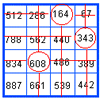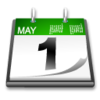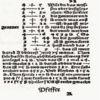#### You may also like### Marvellous Matrix

Follow the directions for circling numbers in the matrix. Add all the circled numbers together. Note your answer. Try again with a different starting number. What do you notice?### On What Day Did it Happen?

Read this article to find out the mathematical method for working out what day of the week each particular date fell on back as far as 1700.### Mathematical Symbols

A brief article written for pupils about mathematical symbols.

# Shapes on the Playground

##### Age 7 to 11 Challenge Level:

Classmates from Moorfield Junior School, Thomas and Robert , explain their strategy for solving some of the challenges of Shapes on the Playground :

To find the shapes that Sally drew they explain:
"We listed all the numbers that add up to 100 and then starting multiplying them by 4 and 8.
The answer we got gave us the solution, 7 squares and 9 octagons.
We then worked Ben's out the same way.
The answer we got gave us the solution 20 squares, 0 triangles and 0 hexagons!!!"

Why do you think Thomas and Robert multiplied by 4 and 8? Do you agree with their answer for the shapes that Ben drew? The problem told us that, "Ben drew triangles, hexagons and squares".

The pupils from St. Aldhelm's C.E. Combined School agree with the solution for Sally, but for Ben they have some other thoughts. They made tables showing the amount of chalk used for each shape and then by completing the tables they discovered how many of each shape Ben would have drawn if he drew 20 shapes in total.

The answer they arrived at was that Ben drew 10 triangles (30 metres), 5 squares (20 metres) and 5 hexagons (30 metres). This used 8 sticks of chalk, as each stick drew 10 metres, leaving two unused sticks. Well done St. Aldhelm's! Can you recognise your initials? LM, ED, RD, HS, KT, DF, LH, NT, AND DR.

Mel and Katie from Loretto Junior School also worked out that Ben could have drawn these shapes:

• 17 squares, 2 triangles, and 1 hexagon
• 8 squares, 8 triangles and 4 hexagons

Very well done!